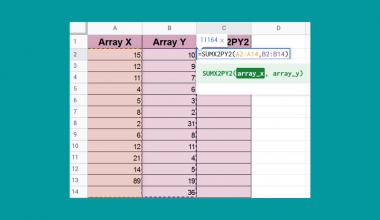# How to Use AVERAGEA Function in Google Sheets

The AVERAGEA function in Google Sheets is used to calculate the numerical average value of a dataset.

`AVERAGEA` is a statistical function that calculates the average value of a group of values. It differs from the `AVERAGE` function, as it also counts in numbers that may have been formatted as text – for example, 1 and 0 as TRUE and FALSE, dates, and so on. The `AVERAGE` function would just ignore these “numbers” otherwise.

This function is useful in the area of statistics.

When might you use the `AVERAGEA` function, among all other types of averages and means? Let’s look at an example.

You are looking for your average score from your semester’s quizzes. Some of the quizzes you missed will be counted as a 0. You have them listed out in a column.

The `AVERAGEA` function is used to get the average or mean of the combined value arguments. In other words, it is the sum of the values of the dataset, divided by the number of values in the dataset.

## The Anatomy of AVERAGEA Function in Google Sheets

The syntax of the `AVERAGEA` function is as follows:

`=AVERAGEA(value1, [value2, ...])`

Let’s have a look at each part of the function to understand what is going on here:

• `=` is the equals sign that starts off any function in Google Sheets.
• `AVERAGEA` is the name of our function.
• `value1` is the first value or range of the dataset.
• `value2, ...` are the additional values or ranges to be included in the dataset. These are optional values.

Note that even with the Google Sheets specification of a maximum of 30 arguments, the function will still support an arbitrary number of arguments.

Again, any text within the dataset will be set to 0, and included in the calculation. If you need to have these value ignored, use the `AVERAGE` function.

## A Real Example of Using AVERAGEA Function

You may be curious as to why the `AVERAGEA` function exists, when there are other ways to calculate averages.

The `AVERAGE` function helps to create correct calculations by including values that seem like text but are actually important to the dataset. This is helpful in a variety of very specific tasks.

Let’s look at the example below to see how to use the `AVERAGEA` function in Google Sheets.

#### Calculating the average in Google Sheets

This is a simple problem. It is a simplified task to help you understand what the function can be used for.In the equation, it will look like this:

```=AVERAGEA(C3,C17)
```

It will result in an average of 61.

While the regular `AVERAGE` calculates it as 70, but this is not completely accurate. The `AVERAGEA` takes note of this and calculates the average properly by including the days where you were absent and thus scored a 0.

This simple problem can be practiced to perfection. Use the link below to get a copy of this problem set:

## How to Use AVERAGEA Function in Google Sheets

In this section, we will show you a step-by-step process on how to use the `HARMEAN` function in Google Sheets.

Another feature of `HARMEAN` is to take of the outliers in a dataset.

In this problem, we are looking at a number of workshop attendees on certain days. We will find the harmonic mean of the attendees and compare it to the average.

#### Using AVERAGEA Function In Google Sheets

1. To begin, prepare your spreadsheet with the information you want to check.1. Start to fill in the `AVERAGEA` function in the cell you want it to appear. In this example, we pick cell F3.1. Start by adding in the `AVERAGEA` function.1. Start by putting in the array of data you want to analyze. You will be able to see a preview.1. You’re done! But now, let’s compare to the regular average.1. You’ll notice that they have significantly different answers to offer, but the harmonic mean treats outliers in a more accurate manner.The `AVERAGEA` function can help make accurate findings from your data.

And there you have it – you can now use the `AVERAGEA` function in Google Sheets together with the other numerous Google Sheets formulas to create even more effective formulas.Our goal this year is to create lots of rich, bite-sized tutorials for Google Sheets users like you. If you liked this one, you'll love what we are working on! Readers receive ✨ early access ✨ to new content. There will be no spam and you can unsubscribe at any time.

##### You May Also Like## How to Use SORT Function in Google Sheets

The SORT function in Google Sheets is useful to sort and return the rows of a range by the…## How to Use SUMX2PY2 Function in Google Sheets

The SUMX2PY2 function in Google Sheets is a mathematical function designed specifically to calculate the sum of the…## How to Calculate Reverse Percentages in Google Sheets

This guide will show you how you can compute reverse percentages in Google Sheets using basic arithmetic operations.…## How to Use IMARGUMENT Function in Google Sheets

You can use the IMARGUMENT function in Google Sheets when you need to know the angle or theta…